# Make Mini UPS For DSL Modem PART-V

This is the fifth post in a series of posts on making your own mini UPS for DSL modem. Be sure to read the first four parts before you read below.

The complete circuit diagram of mini UPS for dsl modem is shown in the Figure 1.Figure 1: Circuit Diagram of Mini UPS For DSL Modem

Click on the image to view enlarged version

## Parts List

You can buy all the parts from online stockists such as Digi-Key USA, Mouser USA, Maplin UK, EVElectronics Pakistan.

Parts List
Reference Description Quantity
Transformer, X1 230V AC Primary to 18V, 2A AC Secondary 1
Fuse 160mA slow-blow 1
Diodes, D1-D7 1N4007 7
Capacitor, C1 4700uF, 50V 1
Capacitor, C2, C7 0.1uF 2
Capacitor, C3 1uF, 50V 1
Capacitor, C4 1uF, 25V 1
Capacitor, C5 100uF, 25V 1
Capacitor, C6 0.33uF, 25V 1
Capacitor, C8 0.01uF 1
Resistor, R1 1.5kΩ, 1/2W 1
Resistor, R2 120Ω, 1/4W 1
Resistor, R3, R4 1.2kΩ, 1/4W 2
Resistor, R5, R8 15kΩ, 1/4W 2
Resistor, R6 180kΩ, 1/4W 1
Resistor, R7 2.2kΩ, 1/4W 1
Resistor, R9 1.5kΩ, 1/4W 1
Resistor, R10A, R10B 1.2Ω, 1/4W 2
Resistor, R11, R12, R1510kΩ, 1/4W 3
Resistor, R13, R16 3.6kΩ, 1/4W 2
Resistor, R14 1.6kΩ, 1/4W 1
Resistor, R17 360Ω, 1/2W 1
Resistor, R18 240Ω, 1/4W 1
Trimpot, VR1 500Ω, 10-turn 1
Trimpot, VR2 5kΩ, 10-turn 1
Trimpot, VR3 100Ω, single-turn 1
Transistor, Q1, Q2 BC548 2
Transistor, Q3 BC337 1
LED1 5mm, Green 1
LED2 5mm, Red 1
IC1 LM317 1
IC2 LM393 1
IC3 NE555 1
IC Socket 8-pin IC Socket 2
Heat Sink less than 5.11 °C/W 1

This circuit diagram of mini UPS for dsl modem is designed to limit the max charge current to 1 A. The charging voltage and float voltage are user adjustable.

## Power Supply

First let us look at the power supply portion. Before we design the power supply we need to work out what voltage and current we want the power supply to deliver. Our DSL modem needs 12V dc, while our battery needs maximum 14.7V dc (remember from part-4 of make mini ups for dsl modem series the maximum voltage, Umax). There are two diodes D5 and D7 between our battery and power supply, each dropping approximately 0.8 V dc. The voltage regulator, LM317 needs minimum 3 V dc (input-to-output differential voltage) to regulate the output voltage properly. Thus we need the power supply to provide a minimum dc voltage of 19.1 V (14.7V + 3V + 0.7V + 0.7V).

The DSL modem which I have draws 230 mA while the recommended constant charging current for my battery is 684 mA. Thus the power supply should be able to deliver at least 914 mA. For simplicity let us make it 1 A.

Thus the power supply should be able to supply 1 A while making sure the supply voltage does not decrease below 19.1 V.

Transformer

A transformer converts high AC voltage from your wall outlet to low valued AC voltage. As seen this is a step down transformer that converts 230V AC to 18V AC. The values are rms values, also known as equivalent dc or effective values. The peak voltage at the secondary side of the transformer will be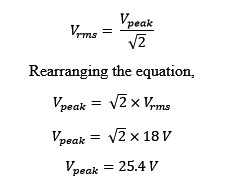Figure 2: Finding the peak value of voltage at the secondary side of the transformer

The manufacturers of the transformers guarantee these values at full load. At no to little load the voltage at the secondary side will be higher than the rated value. For example, the transformer in the circuit diagram has secondary winding rated at 18 Vrms, 2 Arms (rms value of current in secondary winding). Suppose you make the secondary winding open-circuited (means no load) then the voltage across the secondary winding will be greater than 18 Vrms, maybe 20 Vrms. Now if you load the secondary winding at rated 2 Arms current then the voltage across the secondary winding will be no less than 18 V. One should not really load any electronic component more than its rated value. The component may burn or simply activating built-in protection if it is provided.

Bridge Rectifier

The bridge rectifier comprises of diodes D1, D2, D3 and D4. The purpose of the bridge rectifier is to convert AC to DC. We need dc to operate our modem and charge our battery. See Figure 3 to have an idea about what is meant by converting AC to DC.Figure 3: Full wave rectifier
Image by Wdwd/Wikimedia Commons

A full wave rectifier converts ac to dc. The ac has a positive half cycle and a negative half cycle. These two half cycles make a one full cycle. After full wave rectification we get two half cycles which are both positive. The negative half cycle is inverted and becomes the positive half cycle. Thus the dc has only positive voltages.

The process of selection of a diode for a bridge rectifier needs some circuit analysis knowledge. The main parameters to look for are peak repetitive reverse voltage and continuous forward current. The selected diode 1N4007 has peak repetitive reverse voltage of 1000 V and continuous forward current of 1 ampere.

Filter Capacitor

The C1 is a filter capacitor. As seen in the figure 3 the output of the bridge rectifier has lots of variations in it. At one moment the voltage is at its maximum and the next moment it is zero. We need a constant dc voltage with ideally no variations at all. A filter capacitor does this job. It provides nearly constant dc. A capacitor can be thought of as a battery except that it provides stored energy for a very short duration of time.

An easy and reasonably accurate way to size the filtering capacitor is by using the equation of current through a capacitor.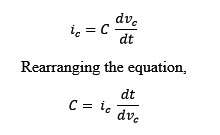Figure 4: Equation of current through a capacitor
where,

C is the capacitance of the capacitor
ic is the current through the capacitor
dt is a very small change in time
dvc is a very small change in voltage

Assume dt to be 10ms (equal to half cycle of ac mains in Asia and Europe) or 8.33ms (equal to half cycle of ac mains in North America) and dvc to be 2.2 V (10% of 22 V). The capacitance is found asFigure 5: Solving for capacitance

The 22 V is the constant dc voltage that I would like the power supply to provide to the rest of the circuit. 2.2 V is the peak to peak value of the ripple. The ripple occurs due to charging and discharging of the capacitor. The ripple is undesirable and one way to reduce ripple is to increase the capacitance of the filtering capacitor.

So we need 4700uF electrolytic capacitor (nearest standard value to 4545uF).

## Battery Charger

Let us now look at battery charger portion.

LM317

LM317 is an adjustable voltage regulator whose output voltage can be fixed at any value from 1.25 V to 37 V. The LM317 can supply a max current of 1.5 A but with sufficient heat sink attached. The three terminal voltage regulator requires only two external resistors to set the output voltage (Figure 6).Figure 6: LM317 needs only two external resistors to set the output voltage

The formula for calculating output voltage is given asFigure 7: Formula for calculating the output voltage of LM317

The current IADJ is typically 50 uA and is negligible in most applications. Thus the formula becomesFigure 8: Formula for calculating the output voltage of LM317 with ADJ current ignored

Vref is typically 1.25 V and this voltage exists between OUTput and ADJust pins. The value of resistor R1 is made fixed while the value of resistor R2 is varied. This allows us to have a variable voltage at the output pin, Vout. Since in the equation, the R2 is in the numerator, thus increasing R2 will increase Vout while decrease in R2 will result in a decrease in output voltage.

As you might have guessed, the LM317 in our circuit of mini UPS allows us to have two different voltages namely charging voltage (14.7 V) and float voltage (13.65 V). As written in the previous post, if a battery is fully charged the battery charger should maintain the voltage across the terminals of the battery at a voltage called float voltage. Now the question is how will the charger detect if the battery is fully charged and how will LM317 decide when to output a charging voltage or float voltage?

This is made possible by a voltage comparator (IC2 LM393) and R5 and VR2. Before understanding this let us first understand how the circuit limits the output current of LM317 to 1 A. When the mains power is available, the output current from LM317 not only charges the battery but also runs the modem simultaneously. And when a battery is fully empty, a very large current follows into the battery that is only limited by a very small internal resistance of the battery. Such high current can damage the battery thus we need to make sure the LM317 in our circuit does not output more than 1 A (in my case my modem will take 230mA. While remaining 770mA will go to the battery, only in the case when the battery is sufficiently discharged. When the battery is partially discharged the current will be lower than 770mA)

The transistor Q1, when turned on, lowers the voltage at the ADJ pin of LM317. This in turn lowers the output voltage of the LM317. As the output voltage drops so will the current and the circuit will “stabilize” to maintain the current limit.

An NPN transistor turns on when the voltage at its base terminal reaches 0.6 V (typically). The resistors R10A and R10B are sized such that the voltage across them is 0.6 V when the current through them is 1 A. A simple application of Ohm’s law gives us this value.

V = IR

Rearranging and putting in values,

A standard fixed resistor does not comes with a resistance of 0.6 Ohm. The lowest standard value is 1 ohm. To have 0.6 ohm resistance, connect two resistors of 1.2 ohm in parallel. When two resistors are in parallel the equivalent resistance is lower than of either resistor. And when two resistors are in series the equivalent resistance is higher than of either resistor.

LM393

The IC2 LM393 consists of two independent voltage comparators. One comparator, IC2: A, is used to set the float voltage while the other, IC2: B, drives the charge indicator LED.

A voltage comparator compares an unknown input voltage with a reference voltage. When the voltage at the positive input of a comparator is higher than the voltage at the negative input then the output of the comparator will be high. When the voltage at the negative input is higher than the voltage at the positive input then the output of the comparator is low.

The reference voltage is set by resistors R6 and R7. This reference voltage varies as the output of the regulator changes. However when the battery is approaching full charge the regulator output will be 15.4 V (14.7 V + 0.7 V across D7). Using the voltage divider rule the reference voltage at pins 2 and 5 of IC2 will be approximately 185mV. While the voltage across the resistors R10A and R10B is more than 185 mV the output of IC2: A will be high (the voltage at negative input is higher than positive input) and R5 and VR2 will not have any effect on the output of the voltage regulator. As the battery reaches full charge the voltage at the positive input of voltage comparator, IC2: A, will be lower than 185 mV making its output low which in turn put R5 and VR2 in parallel with VR1 and R4. This will lower the output voltage of the regulator.

The current required to cause an 185 mV drop across the current limiting resistors R10A and R10B is approximately 308 mA (0.185 V / 0.6 Ohm). Considering the current draw of 230 mA of DSL modem, the charger will switch to float mode when the current into the battery is approximately 78 mA (308mA – 230mA)

The charge indicator LED2 will turn OFF when the battery is fully charged and the charger has switched to float mode.

To set the charging voltage, disconnect the battery and place a digital multimeter instead. Remove the IC2 LM393, then adjust the trimpot VR1 until the multimeter shows 14.7 V. Now insert the IC2 LM393 and adjust the trimpot VR2 until the multimeter shows 13.65 V. Disconnect the power before inserting or removing the IC2.

The IC1 LM317 needs a heatsink because it has to dissipate heat. How to size the heat sink? When do you need a heat sink and when is it not needed?

## Under Voltage Lock Out

The purpose of Under Voltage Lock Out circuitry is to protect the battery from deep discharge. Also called Low Voltage Disconnect, it protects the battery from damage arising from terminal voltage going below 10.5 V. If a battery is being discharged at a slow rate and its terminal voltage falls below 10.5 V, the battery might not recover and may die. So what we need is a mechanism that would not allow the battery terminal voltage to fall below 10.5 V.

Terminal Voltage: The voltage across the battery terminals with the load connected. The terminal voltage varies with the battery's state of charge and charge/discharge current.

Open-circuit Voltage: The voltage across the battery terminals with no load connected. The open-circuit voltage depends on battery’s state of charge, increasing with state of charge.

State of Charge (SoC): The SoC is expressed as present battery capacity as a percentage of maximum battery capacity. The best way to measure SoC is using a hygrometer which is used to check the specific gravity of electrolyte. The sealed lead acid batteries, however, don’t easily lend to this method. There are other ways with the easiest being checking the terminal voltage with a multimeter.

Depth of Discharge (DoD): The battery capacity that has been discharged expressed as a percentage of maximum capacity. A discharge reaching 80% DoD is commonly recognized as a deep discharge.

As an example, if depth of discharge is 40% then the state of charge will be 60%. If the DoD is 80% then SoC will be 20%.

Battery manufacturers provide discharge characteristic curves relating battery terminal voltage with discharge time for various discharge rates. Figure 7 shows the discharge characteristics for the Leoch LPS 12-7.5 battery.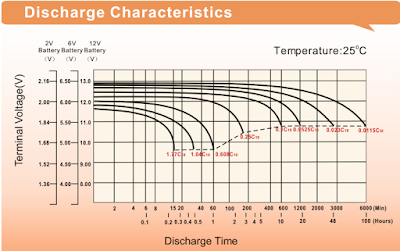Figure 7: Discharge Characteristics of Leoch LPS12-7.5 Battery
Click on the image to view enlarged version.

The figure 7 shows eight discharge characteristic curves for eight different discharge rates. The C10 is the capacity of the battery rated at 10-hr discharge rate. The 10-hr discharge rate means the battery's state of charge will be 0% after 10 hrs of use if you drew a specified current all that time.

The C10 for Leoch LPS12-7.5 is 6.52Ah. The C20 is 6.84Ah. The C100 is 7.5Ah. So drawing 652 mA = 0.652 A for 10 hours will make this battery's state of charge reach 0% (0.652 A * 10 hrs = 6.52Ah).

The battery manufacturers recommend battery's state of charge to be no lower than 50%. Thus, the maximum depth of discharge for a lead acid battery should be no more than 50%. Occasional depth of discharge reaching 60% or 70% is no problem but should not reach 80% at all. Repeatedly making the battery's depth of discharge reach 100% (state of charge 0%) reduces its life.

At what terminal voltage the battery's state of charge is 50%? It is usually around 12 V. A fully charged lead acid battery may show anywhere from 13 to 13.5 V.

Suppose we have a mechanism in place that disconnects the battery from the load when the battery terminal voltage becomes less than 12 V. Further suppose the battery is discharging, its terminal voltage reaches 12 V and then becomes less than 12 V. At this point the UVLO mechanism activates and disconnects the load from the battery. What will happen next is that the battery open-circuit voltage will jump up becoming more than 12 V and thus reconnecting the load with the battery. This repeated on/off oscillatory behaviour is obviously bad and should be avoided.

The solution is to select a lower threshold for disconnecting the load and an upper threshold for reconnecting the load. The difference between these quantities should be greater than the amount by which the battery voltage jumps up.

If we disconnect the load from the battery when its voltage is X, we only re-connect it if the battery voltage has increased to X + y (where y is greater than the jump in the battery voltage when the load disconnects). For 12V lead acid batteries we have found that the value of y should be at least 0.5V, and ideally 1.0V for greater system stability.
Source.

In electronics the difference between the lower threshold and upper threshold is called hysteresis. In the figure 8, the 12.0 V is the lower threshold, the 13.4 V is the upper threshold, and the hysteresis is 1.4 V (13.4V - 12V).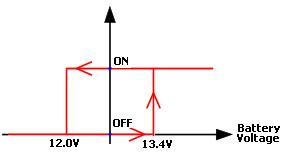Figure 8: Hysteresis in Low Voltage Disconnect

One way to implement under voltage lock out mechanism with hysteresis is by using a NE555 timer. The design calls for a single integrated circuit – the NE555 timer IC to accomplish the tasks such as measuring battery terminal voltage, outputting the relevant signal. The figure 9 shows the block diagram of NE555.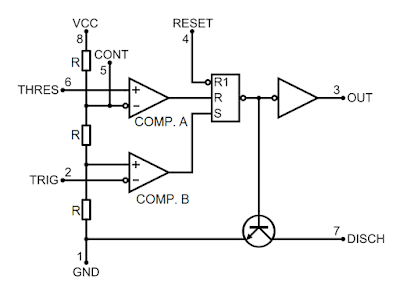Figure 9: NE555 Block Diagram

The three resistors in the block diagram above are of equal value of 5 kΩ. These three resistors make a voltage divider. The voltage at the negative input of comparator A is 2/3 of Vcc with respect to GND. The voltage at the positive input of comparator B is 1/3 of Vcc with respect to GND.

When the trigger input goes below 1/3 Vcc, the output of comparator B becomes HIGH and sets the latch. This causes the output pin to go and stay HIGH.

When the threshold input goes above 2/3 Vcc, the output of comparator A becomes LOW and resets the latch. This causes the output pin to go and stay LOW.

Here is a brief explanation of under voltage lock portion of the circuit diagram of mini UPS.

The LM7805 supplies a fixed 5V to the NE555 chip. Therefore the trigger input is waiting to respond to a voltage of less than 1/3 of 5V = 1.667V, and the threshold input is waiting to respond to a voltage of greater than 2/3 of 5V = 3.333V. So, we need to set the resistor values in the voltage dividers so that the trigger pin will see 1.667V when the battery voltage is at the voltage we want to engage the low voltage disconnect, and the threshold pin will see 3.333V when the battery voltage is at the voltage we want to cancel the low voltage disconnect.
Source.

In the circuit I’ve selected the values for R13 and R14 such that the when the battery terminal voltage is less than 12 V the DSL modem will turn off. When the modem has turned off, it will turn on again only when the battery terminal voltage has risen to 12.8 V. Follow this link to find how to select resistor values in case you want different lower and upper thresholds.

The variable resistor VR3 is not there to have a different lower threshold. To set the lower threshold at 12 V, the R14 should have exactly 1.6133 kΩ (rounded to four decimal places) resistance. The nearest standard value is 1.6 kΩ. The resistors come in different tolerances, +-1%, +-5%, +-20%. If you can get the resistor with +-1% tolerance, you don’t need the VR3. If only +-5% tolerance resistors are available then use VR3 and adjust accordingly to have a lower threshold at precisely 12 V.

To turn on the modem the output of NE555 should be LOW while for turning it off the output should be HIGH.

The transistor Q2 and resistors R16, R17 collectively work as an inverter. An inverter inverts logic LOW at its input to logic HIGH at its output and vice versa.

The transistor Q3 and resistor R18 together act as a switch.

The push button switch is there to help you when you really need to turn on the modem when it has automatically turned off due to low battery. Pressing it will reset the latch inside NE555 and hence the output pin will go LOW turning on the modem.

One important thing to note is that your modem should be able to take 14.7 V. When the modem is ON it is effectively in parallel with the battery. Thus the 14.7 V, which are meant for modem, also come across the modem. If your modem is not too old, chances are it will work fine. However, if you don’t want to take risk, use a low drop voltage regulator like LM2940.

I hope you have understood everything. Still, if there is something you don't understand or need further explanation, feel free to ask in comments below. :)

Unknown said...

Hi adil akbar brother , how are you ?
Its a very nice project with explanation , i have completed your project for myselft and it works ,
And i am thankful for you.
But my requirments sonething different , my tp-link router(9v,600amp max) and dsl or midea converter (12v,1amp max) ,so i need another 9 volt extra supply from this citcuit
1) how to make 9 v supply from this citcuit ?
2) also i want 5 v supply at a time how it would be?
3) u made this project for u so i want to know is still ur ups provides good survice?? Or what u had to changeover on this circuit?
Thank you brother for everything

Regards ,
Nazmul Hasan